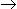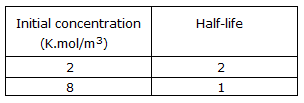# Chemical Engineering - Chemical Reaction Engineering - Discussion

1.

The following half life data are available for the irreversible liquid phase reaction Aproducts.The overall order of reaction is

 [A]. 0.5 [B]. 1 [C]. 1.5 [D]. 2

Explanation:

No answer description available for this question.

 Abere said: (Aug 16, 2018) Please describe the solution clearly.

 Udaya said: (Mar 18, 2019) Answer should be 2.

 Aadhar Chauhan said: (Feb 3, 2020) t(1/2) is directly proportional to (initial conc.)^(1-n). n= Order of Reaction; Hence 2/1=(2/8)^(1-n), Take Log on both side then; ln(2)=(1-n)*(ln(0.25)). So On Solving; n=1.5.